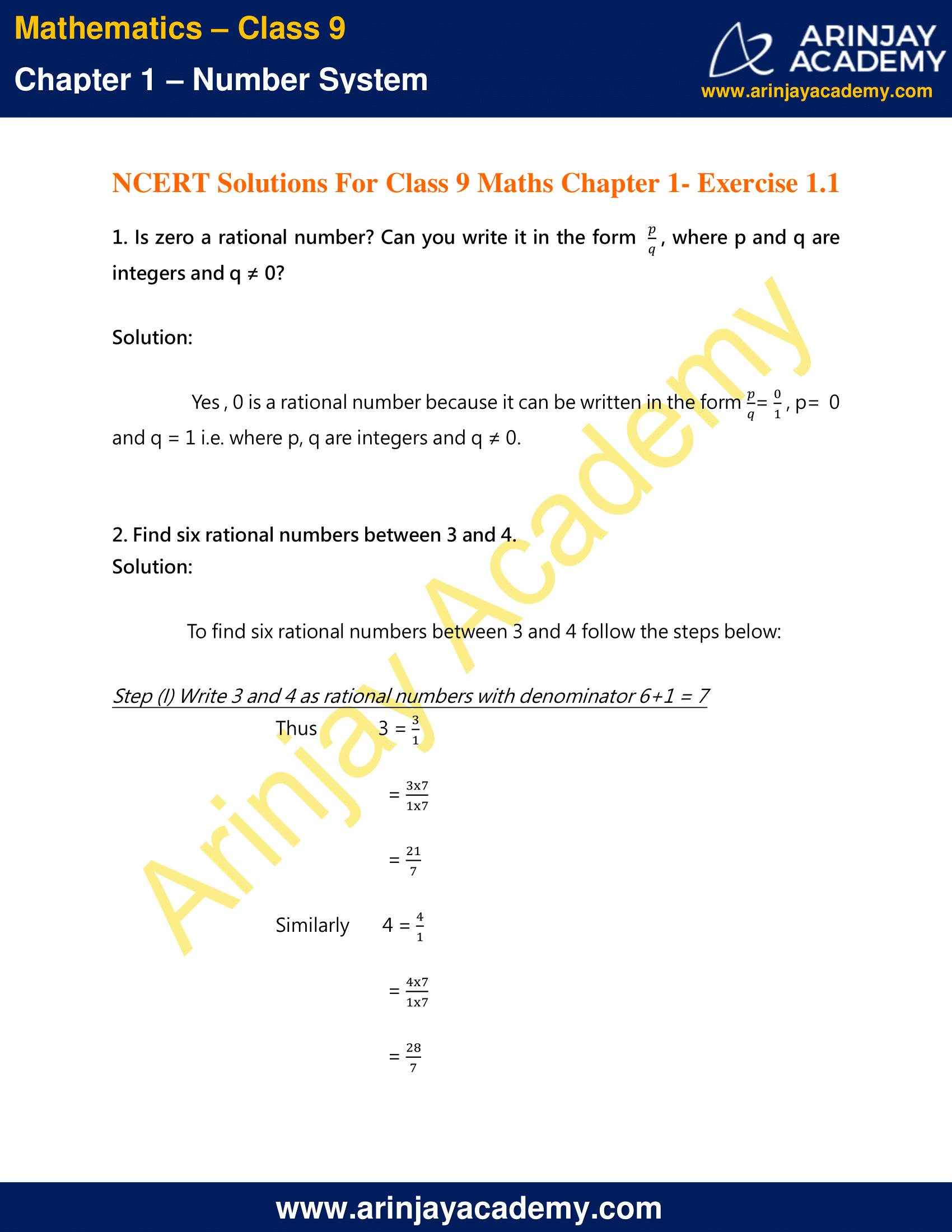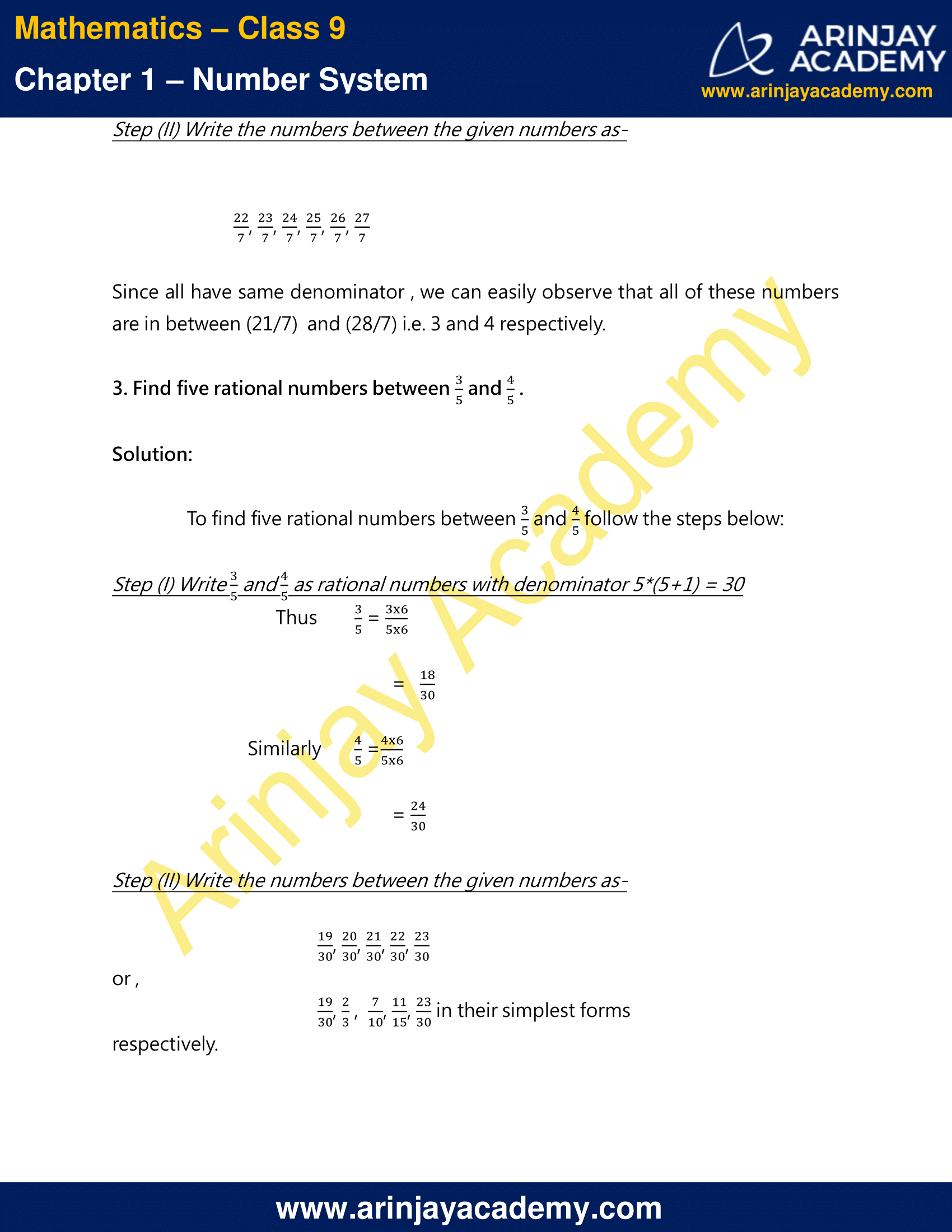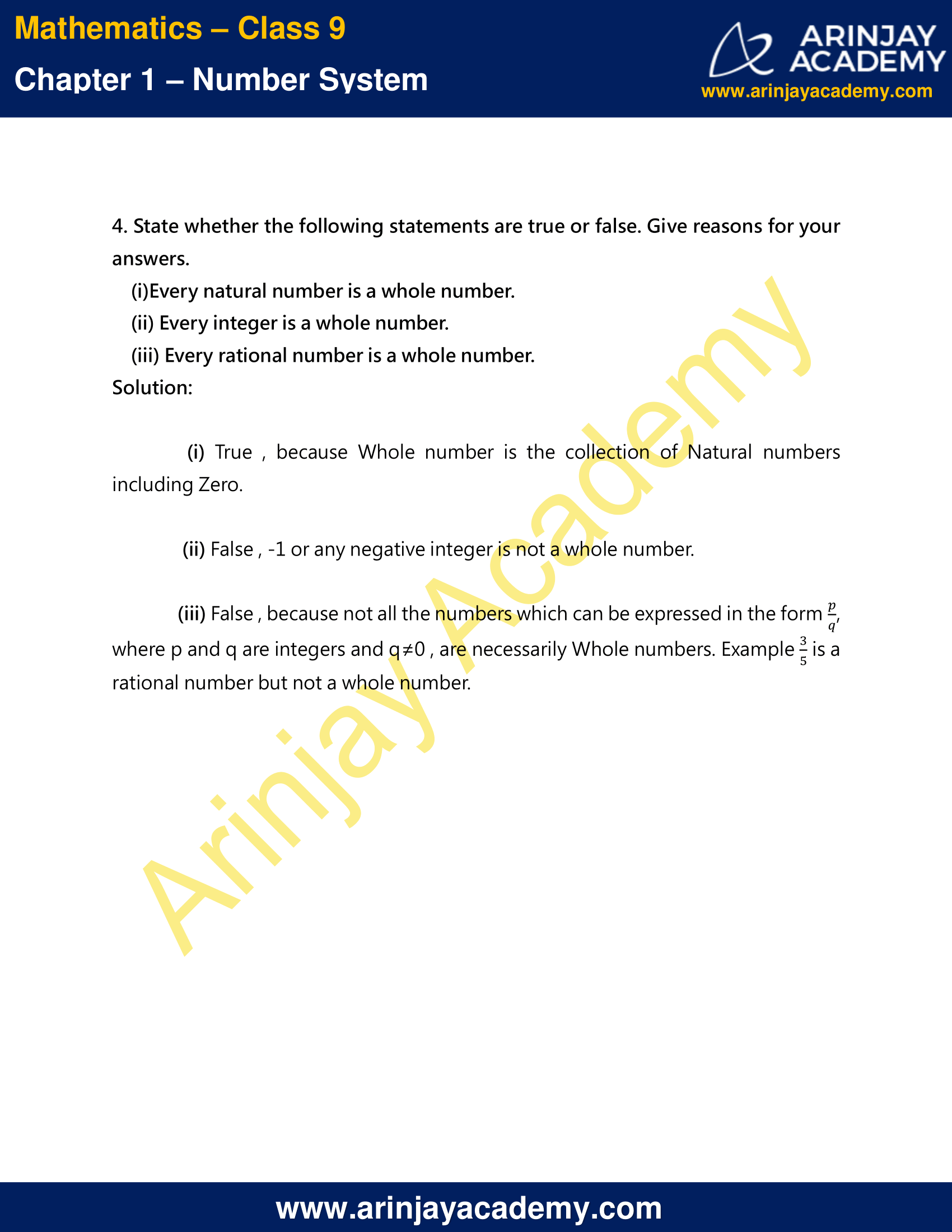# NCERT Solutions For Class 9 Maths Chapter 1 Exercise 1.1 – Number System

Download NCERT Solutions For Class 9 Maths Chapter 1 Exercise 1.1 – Number System. This Exercise contains 4 questions, for which detailed answers have been provided in this note. In case you are looking at studying the remaining Exercise for Class 9 for Maths NCERT solutions for Chapter 1 or other Chapters, you can click the link at the end of this Note.

### NCERT Solutions For Class 9 Maths Chapter 1 Exercise 1.1 – Number SystemNCERT Solutions For Class 9 Maths Chapter 1 Exercise 1.1 – Number System

### What is a Rational Number – Video Explanation

1. Is zero a rational number? Can you write it in the form p/q, where p and q are integers
and q ≠ 0?

Solution 1

Yes , 0 is a rational number because it can be written in the form p/q = 0/1, p= 0 and q = 1 i.e. where p, q are integers and q ≠ 0 .

### Finding Rational Numbers between two Rational Numbers – Video Explanation

2. Find six rational numbers between 3 and 4.

Solution 2

To find six rational numbers between 3 and 4 follow the steps below:

Step (I) Write 3 and 4 as rational numbers with denominator 6+1 = 7

Thus, 3 = 3/1

= (3 x 7)/(1 x 7)

= 21/7

Similarly, 4 = 4/1

= (4 x 7)/(1 x 7)

= 28/7

Step (II) Write the numbers between the given numbers as-

22/7, 23/7, 24/7, 25/7, 26/7, 27/7

Since all have same denominator , we can easily observe that all of these numbers are in between 21/7 and 28/7 i.e. 3 and 4 respectively.

3. Find five rational numbers between 3/5 and 4/5.

Solution 3

To find five rational numbers between 3/5 and 4/5 follow the steps below:

Step (I) Write 3/5 and 4/5 as rational numbers with denominator 5 x (5+1) = 30
Thus 3/5 = (3 x 6)/(5 x 6) = 18/30

Similarly, 4/5 = (4 x 6)/(5 x 6) = 24/30

Step (II) Write the numbers between the given numbers as-

19/30, 20/30, 21/30, 22/30, 23/30

or , 19/30, 2/3, 7/30, 11/15, 23/30 in their simplest forms respectively.

4. State whether the following statements are true or false. Give reasons for your answers.
(i)Every natural number is a whole number.
(ii) Every integer is a whole number.
(iii) Every rational number is a whole number.

Solution 4

(i) True , because Whole number is the collection of Natural numbers including Zero.
(ii) False , -1 or any negative integer is not a whole number.
(iii) False , because not all the numbers which can be expressed in the form p/q, where p and q are integers and q≠0 , are necessarily Whole numbers. Example 3/5 is a rational number but not a whole number.

NCERT Solutions for Class 9 Maths Chapter 1 Exercise 1.1 – Number System, has been designed by the NCERT to test the knowledge of the student on the topic – Introduction of Number System

The next Exercise for NCERT Solutions for Class 9 Maths Chapter 1 Exercise 1.2 – Number System can be accessed by clicking here.

Download NCERT Solutions For Class 9 Maths Chapter 1 Exercise 1.1 – Number System